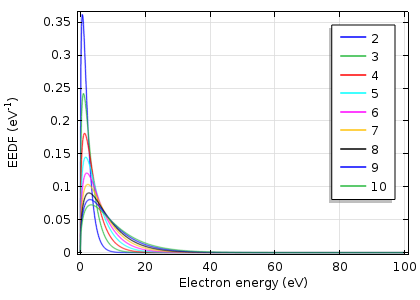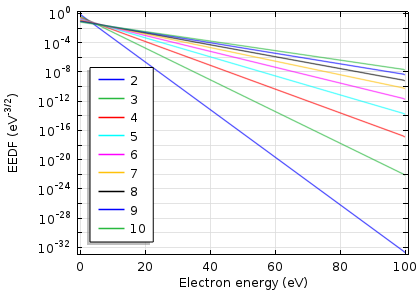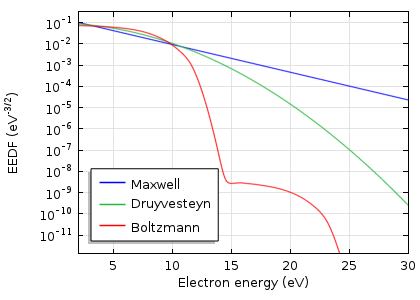# 电子能量分布函数

2014年 8月 4日

### 描述 EEDF

#### 描述 EEDF

 麦克斯韦 f(\epsilon)=\varphi^{-3/2}\beta_1\exp\left(-\frac{\epsilon\beta_2}{\varphi}\right) \beta_1=\Gamma(5/2)^{3/2}\Gamma(3/2)^{-5/2},\ \beta_2=\Gamma(5/2)\Gamma(3/2)^{-1} Druyvesteyn f(\epsilon)=\varphi^{-3/2}\beta_1\exp\left(-\left(\frac{\epsilon\beta_2}{\varphi}\right)^2\right) \beta_1=\Gamma(5/4)^{3/2}\Gamma(3/4)^{-5/2},\ \beta_2=\Gamma(5/4)\Gamma(3/4)^{-1} 广义形式 f(\epsilon)=\varphi^{-3/2}\beta_1\exp\left(-\left(\frac{\epsilon\beta_2}{\varphi}\right)^g\right) \beta_1=\Gamma(5/2g)^{3/2}\Gamma(3/2g)^{-5/2},\ \beta_2=\Gamma(5/2g)\Gamma(3/2g)^{-1}

#### Druyvesteyn 函数

Druyvesteyn EEDF 是基于一个恒定的(与电子能量无关的)横截面，麦克斯韦 EEDF 是基于恒定的碰撞频率。分布函数假定弹性碰撞占主导地位，因此非弹性碰撞(如激发或电离)对分布函数的影响不显著。在这种情况下，分布函数变为球对称。在与中性原子的弹性碰撞中，电子的运动方向改变了，但它们的能量(由于巨大的质量差异)不变。

#### 玻耳兹曼方程

\frac{\partial f}{\partial t}+\mathbf{v}\cdot\triangledown f-\frac{e}{m}(\mathbf{E}\cdot\triangledown_\mathbf{v}f)=C[f]### 比较麦克斯韦、Druyvesteyn 和波尔兹曼分布函数

Druyvesteyn 分布的最大和平均能量会转移到更高的值。与麦克斯韦分布相比，高能尾下降得更快。当电子达到足以激发或电离的能量时，就会发生非弹性碰撞。这导致玻尔兹曼分布函数的下降，如下所示。### 影响模型结果

k_k=\gamma\int\limits_{0}^{\infty}\epsilon\sigma_k(\epsilon)f(\epsilon)\mathrm d\epsilon### 介质阻挡放电模型结果的比较DBD 中总放电电流与时间的关系。DBD 吸收的功率与时间的关系。